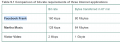# Calculation of bit rate and byte transferred

#### zulfi100

Joined Jun 7, 2012
617
Hi,
A book shows some data and then it calculates the bit rate and byes transferred in 4000s. The text is:

Let’s assume
that Frank is looking at a new photo every 10 seconds, and that photos are on average 200 Kbytes in
size. (As usual, throughout this discussion we make the simplifying assumption that )
Our second user, Martha, is streaming music from the Internet (“the cloud”) to her smartphone. Let’s
assume Martha is using a service such as Spotify to listen to many MP3 songs, one after the other,
each encoded at a rate of 128 kbps. Our third user, Victor, is watching a video that has been encoded at
2 Mbps. Finally, let’s suppose that the session length for all three users is 4,000 seconds (approximately
67 minutes).

Using the above information author constructs the table attached.

Some body please guide me how the table is constructed?

Zulfi.

#### ci139

Joined Jul 11, 2016
797
transfer protocols use data stream formats , device addressing ← that add some bytes to data being sent // and then (perhaps) a Live compression ← the last is quite hard to predict e.g. the non-compressible files such as .jpg .mp3 won't get much optimized by size while .html .txt may get compressed below 5% of the original

Last edited:

#### WBahn

Joined Mar 31, 2012
24,854
size. (As usual, throughout this discussion we make the simplifying assumption that )
simplifying assumption that ..... what?????

Don't make us guess all the time!

If data is being transferred at 128 kbps, how many bits are transferred in 4000 seconds? How many MB is this?

#### WBahn

Joined Mar 31, 2012
24,854
transfer protocols use data stream formats , device addressing ← that add some bytes to data being sent // and then (perhaps) a Live compression ← the last is quite hard to predict e.g. the non-compressible files such as .jpg .mp3 won't get much optimized by size while .html .txt may get compressed below 5% of the original
Problems at this introductory level almost always ignore such issues.

•ci139

#### zulfi100

Joined Jun 7, 2012
617
simplifying assumption that ..... what?????

Don't make us guess all the time!

If data is being transferred at 128 kbps, how many bits are transferred in 4000 seconds? How many MB is this?
In 1s - 128 kb transferred
in 4000s- 128 * 4000 = 512000 K bits
1MB = 1024 * 1024 bytes

Now 512000K bits = 512000 * 1024 * bits
= (512000 * 1024 )/ (1024 * 1024) M bits
= 500 M bits
= (500/8) MBytes
= 62.5 MB (This should be 64MB or 63.5???)

Now for Victor 2 Mb/s , so in 4000s = 8000Mb = 1000 Mbytes
Now 1 GB = 1024 M
Therefore 1000Mbytes/(1024 M)
= 1 GB (Not perfect)

Zulfi.

#### WBahn

Joined Mar 31, 2012
24,854
Again, what is that simplifying assumption that the problem states is usually made?

Could it be that 2^10 ~= 1000?